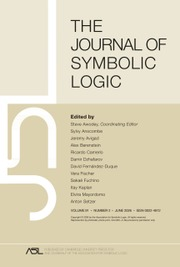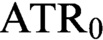Home
Hostname: page-component-768dbb666b-v9bzm Total loading time: 0.851 Render date: 2023-02-06T03:08:02.886Z Has data issue: true Feature Flags: { "useRatesEcommerce": false } hasContentIssue trueThe Journal of Symbolic Logic

# SEARCHING FOR AN ANALOGUE OF ATR0 IN THE WEIHRAUCH LATTICE

Published online by Cambridge University Press:  10 July 2020

## Abstract

There are close similarities between the Weihrauch lattice and the zoo of axiom systems in reverse mathematics. Following these similarities has often allowed researchers to translate results from one setting to the other. However, amongst the big five axiom systems from reverse mathematics, so far$\mathrm {ATR}_0$ has no identified counterpart in the Weihrauch degrees. We explore and evaluate several candidates, and conclude that the situation is complicated.

## MSC classification

Type
Articles
Information
The Journal of Symbolic Logic , September 2020 , pp. 1006 - 1043
© The Association for Symbolic Logic 2020

## Access options

Get access to the full version of this content by using one of the access options below. (Log in options will check for institutional or personal access. Content may require purchase if you do not have access.)

## References

#### REFERENCES

Bienvenu, L., Greenberg, N., and Monin, B., Continuous higher randomness . Journal of Mathematical Logic , vol. 17 (2017), no. 1, p. 53.CrossRefGoogle Scholar
Blass, A., Complexity of winning strategies . Discrete Mathematics , vol. 3 (1972), pp. 295300.CrossRefGoogle Scholar
Brattka, V., Effective borel measurability and reducibility of functions . Mathematical Logic Quarterly , vol. 51 (2005), pp. 1944.CrossRefGoogle Scholar
Brattka, V., A Galois connection between turing jumps and limits . Logical Methods in Computer Science , vol. 14 (2018), no. 3, pp. 137.Google Scholar
Brattka, V., de Brecht, M., and Pauly, A., Closed choice and a uniform low basis theorem . Annals of Pure and Applied Logic , vol. 163 (2012), no. 8, pp. 9681008.CrossRefGoogle Scholar
Brattka, V. and Gherardi, G., Effective choice and boundedness principles in computable analysis . Bulletin of Symbolic Logic , vol. 17 (2011), pp. 73117.CrossRefGoogle Scholar
Brattka, V. and Gherardi, G., Weihrauch degrees, omniscience principles and weak computability, this Journal, vol. 76 (2011), pp. 143176.Google Scholar
Brattka, V., Gherardi, G., and Hölzl, R., Probabilistic computability and choice . Information and Computation , vol. 242 (2015), pp. 249286.CrossRefGoogle Scholar
Brattka, V., Gherardi, G., Hölzl, R., Nobrega, H., and Pauly, A., Borel choice, in preparation.Google Scholar
Brattka, V., Gherardi, G., Hölzl, R., and Pauly, A., The Vitali covering theorem in the Weihrauch lattice , Computability and Complexity: Essays Dedicated to Rodney G. Downey on the Occasion of His 60th Birthday (Day, A., Fellows, M., Greenberg, N., Khoussainov, B., Melnikov, A., and Rosamond, F., editors), Springer International Publishing, Cham, 2017, pp. 188200.CrossRefGoogle Scholar
Brattka, V., Gherardi, G., and Marcone, A., The Bolzano-Weierstrass Theorem is the jump of Weak König’s Lemma . Annals of Pure and Applied Logic , vol. 163 (2012), no. 6, pp. 623625.CrossRefGoogle Scholar
Brattka, V., Gherardi, G., and Pauly, A.. Weihrauch complexity in computable analysis. Preprint, 2017. http://arxiv.org/abs/1707.03202.Google Scholar
Brattka, V., Kawamura, A., Marcone, A., and Pauly, A., Measuring the complexity of computational content (Dagstuhl Seminar 15392) . Dagstuhl Reports , vol. 5 (2016), no. 9, pp. 77104.Google Scholar
Brattka, V. and Pauly, A., Computation with advice . Electronic Proceedings in Theoretical Computer Science , vol. 24 (2010), pp. 4155 CrossRefGoogle Scholar
Brattka, V. and Pauly, A., On the algebraic structure of Weihrauch degrees . Logical Methods in Computer Science , vol. 14 (2018), no. 4, pp. 136 Google Scholar
Chong, C. T. and Yu, L., Randomness in the higher setting , this Journal, vol. 80 (2015), no. 4, pp. 11311148.Google Scholar
Dzhafarov, D., Joins in the strong Weihrauch degrees . Mathematical Research Letters , vol. 26 (2019), no. 3, pp. 749767.CrossRefGoogle Scholar
Dzhafarov, D. D., Le Goh, J., Hirschfeldt, D. R., Patey, L., and Pauly, A., Ramsey’s theorem and products in the Weihrauch degrees. Preprint, 2018. https://arxiv.org/abs/1804.10968.Google Scholar
Friedman, H. M. and Hirst, J. L., Weak comparability of well orderings and reverse mathematics . Annals of Pure and Applied Logic , vol. 47 (1990), pp. 1129.CrossRefGoogle Scholar
Gherardi, G. and Marcone, A., How incomputable is the separable Hahn-Banach theorem? Notre Dame Journal of Formal Logic , vol. 50 (2009), no. 4, pp. 393425.CrossRefGoogle Scholar
Goh, J. L., Embeddings between well-orderings: computability-theoretic reductions . Annals of Pure and Applied Logic , vol. 171 (2020), no. 6, art. 102789.CrossRefGoogle Scholar
Gregoriades, V., Kispéter, T., and Pauly, A., A comparison of concepts from computable analysis and effective descriptive set theory . Mathematical Structures in Computer Science , vol. 27 (2017), vol. 8, pp. 14141436.CrossRefGoogle Scholar
Higuchi, K. and Pauly, A., The degree-structure of Weihrauch-reducibility . Logical Methods in Computer Science , vol. 9 (2013), no. 2, pp. 117.CrossRefGoogle Scholar
Hirschfeldt, D. R., Slicing the Truth: On the Computability Theoretic and Reverse Mathematical Analysis of Combinatorial Principles , World Scientific, Hackensack, NJ, 2014.CrossRefGoogle Scholar
Jockusch, C. G. Jr. and Soare, R. I.,${\varPi}_1^0$ classes and degrees of theories . Transactions of the American Mathematical Society , vol. 173 (1972), pp. 3356.Google Scholar
Kihara, T. and Pauly, A., Dividing by zero – How bad is it, really? 41st International Symposium on Mathematical Foundations of Computer Science (MFCS 2016) (Faliszewski, P., Muscholl, A., and Niedermeier, R., editors), Leibniz International Proceedings in Informatics (LIPIcs), volume 58, Schloss Dagstuhl, Dagstuhl, 2016, pp. 58:158:14.Google Scholar
Kleene, S. C., Hierarchies of number-theoretic predicates . Bulletin of the American Mathematical Society , vol. 61 (1955), pp. 193213.CrossRefGoogle Scholar
Kleene, S. C., Quantification of number-theoretic functions . Compositio Mathematica , vol. 14 (1959), pp. 2340.Google Scholar
Kreisel, G., Analysis of the Cantor-Bendixson theorem by means of the analytic hierarchy . Bulletin de l’Académie Polonaise des Sciences. Série des Sciences Mathématiques, Astronomiques et Physiques , vol. 7 (1959), pp. 621626.Google Scholar
Le Roux, S. and Pauly, A., Weihrauch degrees of finding equilibria in sequential games , Evolving Computability (Beckmann, A., Mitrana, V., and Soskova, M., editors), Lecture Notes in Computer Science, volume 9136, Springer, Cham, 2015, pp. 246257.CrossRefGoogle Scholar
Montalbán, A., On the${\varPi}_1^1$ -separation principle . Mathematical Logic Quarterly , vol. 54 (2008), no. 6, pp. 563578.CrossRefGoogle Scholar
Neumann, E. and Pauly, A., A topological view on algebraic computations models . Journal of Complexity , vol. 44 (2018), pp. 122.CrossRefGoogle Scholar
Nies, A., Computability and Randomness , Oxford Logic Guides, volume 51, Oxford, Oxford University Press, 2009.CrossRefGoogle Scholar
Nobrega, H. and Pauly, A., Game characterizations and lower cones in the Weihrauch degrees . Logical Methods in Computer Science , vol. 15 (2019), no. 3, pp. 11.111.29.Google Scholar
Pauly, A., How incomputable is finding Nash equilibria? Journal of Universal Computer Science , vol. 16 (2010), no. 18, pp. 26862710.Google Scholar
Pauly, A., On the (semi)lattices induced by continuous reducibilities . Mathematical Logic Quarterly , vol. 56 (2010), no. 5, pp. 488502.CrossRefGoogle Scholar
Pauly, A., Computable metamathematics and its application to game theory , Ph.D. thesis, University of Cambridge, 2012.Google Scholar
Pauly, A., On the topological aspects of the theory of represented spaces . Computability , vol. 5 (2016), no. 2, pp. 159180.CrossRefGoogle Scholar
Pauly, A., Computability on the space of countable ordinals, this Journal, accepted for publication. http://arxiv.org/abs/1501.00386.Google Scholar
Pauly, A. and de Brecht, M., Towards synthetic descriptive set theory: An instantiation with represented spaces. Preprint, 2013. http://arxiv.org/abs/1307.1850.Google Scholar
Sacks, G. E., Higher Recursion Theory , Perspectives in Mathematical Logic, vol. 2, Springer-Verlag, Berlin, 1990.CrossRefGoogle Scholar
Simpson, S., Subsystems of Second Order Arithmetic , Perspectives in Logic, Cambridge University Press, Cambridge, 2009.CrossRefGoogle Scholar
von Stein, T., Vergleich nicht konstruktiv lösbarer Probleme in der Analysis , Diplomarbeit , Fachbereich Informatik, FernUniversität Hagen, 1989.Google Scholar
Weihrauch, K., The degrees of discontinuity of some translators between representations of the real numbers , Informatik Berichte , vol. 129, FernUniversität Hagen, Hagen, 1992.Google Scholar
4
Cited by

# Save article to Kindle

Note you can select to save to either the @free.kindle.com or @kindle.com variations. ‘@free.kindle.com’ emails are free but can only be saved to your device when it is connected to wi-fi. ‘@kindle.com’ emails can be delivered even when you are not connected to wi-fi, but note that service fees apply.

Find out more about the Kindle Personal Document Service.

SEARCHING FOR AN ANALOGUE OF ATR0 IN THE WEIHRAUCH LATTICE
Available formats
×

# Save article to Dropbox

To save this article to your Dropbox account, please select one or more formats and confirm that you agree to abide by our usage policies. If this is the first time you used this feature, you will be asked to authorise Cambridge Core to connect with your Dropbox account. Find out more about saving content to Dropbox.

SEARCHING FOR AN ANALOGUE OF ATR0 IN THE WEIHRAUCH LATTICE
Available formats
×

# Save article to Google Drive

To save this article to your Google Drive account, please select one or more formats and confirm that you agree to abide by our usage policies. If this is the first time you used this feature, you will be asked to authorise Cambridge Core to connect with your Google Drive account. Find out more about saving content to Google Drive.

SEARCHING FOR AN ANALOGUE OF ATR0 IN THE WEIHRAUCH LATTICE
Available formats
×
×